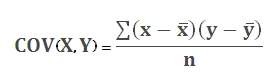# SQL Server covariance function

Updated: 6 August 2010

Use COVAR_q to calculate the average of the products of the deviations for each data point pair. The equation for covariance isSyntax
SELECT [wctStatistics].[wct].[COVAR_q] (
<@Known_y_Known_x_RangeQuery, nvarchar(4000),>)
Arguments
@Known_y_known_x_RangeQuery
the select statement, as text, used to determine the known y- and x-values to be used in the COVAR_q calculation.
Return Types
float
Remarks
·         If the number of known-x data points is different than the number of known-y data points, COVAR_q will return an error.
·         If the standard deviation of either set of data points is zero, then COVAR_q will return an error.
·          This function works with normalized and de-normalized tables.
·         No GROUP BY is required for this function even though it produces aggregated results.
Examples
To determine the covariance between grades in math and grades in science on a normalized table:
from #s1 a, #s1 b
where a.subject = ' + Char(39) + 'Math' + Char(39) + ' and
b.subject = ' + Char(39) + 'Science' + Char(39) + 'and
a.student = b.student')

This produces the following result

----------------------
13.7518590909091

(1 row(s) affected)

To determine the covariance between grades in math and grades in science on a de-normalized table:

SELECT wct.COVAR_q('SELECT math, science from #s2')

This produces the following result

----------------------
13.7518590909091

(1 row(s) affected)

### SupportCopyright 2008-2023 Westclintech LLC         Privacy Policy        Terms of Service